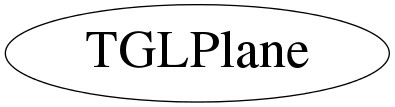# class TGLPlane

```
TGLPlane

3D plane class - of format Ax + By + Cz + D = 0

This is part of collection of simple utility classes for GL only in
TGLUtil.h/cxx. These provide const and non-const accessors Arr() &
CArr() to a GL compatible internal field - so can be used directly
with OpenGL C API calls - which TVector3 etc cannot (easily).
They are not intended to be fully featured just provide minimum
required.

```

## Function Members (Methods)

public:
 TGLPlane() TGLPlane(const TGLPlane& other) TGLPlane(Double_t* eq) TGLPlane(const TGLVector3& norm, const TGLVertex3& point) TGLPlane(const TGLVertex3& p1, const TGLVertex3& p2, const TGLVertex3& p3) TGLPlane(Double_t a, Double_t b, Double_t c, Double_t d) virtual ~TGLPlane() Double_t A() const Double_t* Arr() Double_t B() const Double_t C() const const Double_t* CArr() const static TClass* Class() Double_t D() const Double_t DistanceTo(const TGLVertex3& vertex) const void Dump() const virtual TClass* IsA() const TGLVertex3 NearestOn(const TGLVertex3& point) const void Negate() TGLVector3 Norm() const TGLPlane& operator=(const TGLPlane&) void Set(const TGLPlane& other) void Set(Double_t* eq) void Set(const TGLVector3& norm, const TGLVertex3& point) void Set(const TGLVertex3& p1, const TGLVertex3& p2, const TGLVertex3& p3) void Set(Double_t a, Double_t b, Double_t c, Double_t d) virtual void ShowMembers(TMemberInspector&) virtual void Streamer(TBuffer&) void StreamerNVirtual(TBuffer& ClassDef_StreamerNVirtual_b)
private:
 void Normalise()

## Data Members

private:
 Double_t fVals

## Class Charts## Function documentation

TGLPlane()
``` Construct a default plane of x + y + z = 0
```
TGLPlane(const TGLPlane& other)
``` Construct plane from 'other'
```

``` Construct plane with equation a.x + b.y + c.z + d = 0
with optional normalisation
```
TGLPlane(Double_t* eq)
``` Construct plane with equation eq.x + eq.y + eq.z + eq = 0
with optional normalisation
```
TGLPlane(const TGLVertex3& p1, const TGLVertex3& p2, const TGLVertex3& p3)
``` Construct plane passing through 3 supplied points
with optional normalisation
```
TGLPlane(const TGLVector3& norm, const TGLVertex3& point)
``` Construct plane with supplied normal vector, passing through point
with optional normalisation
```

``` Destroy plane object
```
void Normalise()
``` Normalise the plane.
```
void Dump() const
``` Output plane equation to std::out
```
void Set(const TGLPlane& other)
``` Assign from other.
```
void Set(Double_t a, Double_t b, Double_t c, Double_t d)
``` Set by values.
```
void Set(Double_t* eq)
``` Set by array values.
```
void Set(const TGLVector3& norm, const TGLVertex3& point)
``` Set plane from a normal vector and in-plane point pair
```
void Set(const TGLVertex3& p1, const TGLVertex3& p2, const TGLVertex3& p3)
``` Set plane by three points.
```
void Negate()
``` Negate the plane.
```
Double_t DistanceTo(const TGLVertex3& vertex) const
``` Distance from plane to vertex.
```
TGLVertex3 NearestOn(const TGLVertex3& point) const
``` Return nearest point on plane.
```
const Double_t * CArr() const
`{ return fVals; }`
Double_t * Arr()
`{ return fVals; }`
Double_t A() const
``` Accessors
```
`{ return fVals; }`
Double_t B() const
`{ return fVals; }`
Double_t C() const
`{ return fVals; }`
TGLVector3 Norm() const
`{ return TGLVector3( fVals, fVals, fVals); }`
TGLColor& operator=(const TGLPlane& )